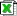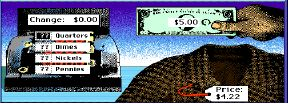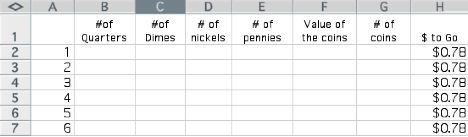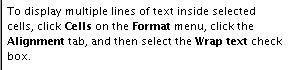Lesson: The Boutique Shop
 Student Teacher Extensionsboutique.xls In the Math Shop (1998) version of Boutique the student plays the role of a store clerk who sells clothes. The main task is to handle the exchange of money and give correct change. In this example, the student is given \$5.00 for an item that costs \$4.22. The right change is 78¢.Assuming you have at least 9 of each coin (quarters, dimes, nickels and pennies) in your register, what are the different ways you could make the necessary change? What's the minimum number of coins that would make this change? What's the maximum number of coins that make this change? Remember: You can't use more than 9 of any particular coin. A spreadsheet is a handy tool to help you with this problem. Open a new Excel worksheet (or use the Boutique Spreadsheet Template.) Set up a spreadsheet that looks like this.In order for the spreadsheet to do the calculations for you, you will need formulas to find the value of the coins, the total number of coins, and the amount of money that you still need to give the customer. (This last column is not absolutely necessary but is very helpful.) The formula: "behind" cell F2 is: =B2*.25 + C2*.10 + D2*.05 + E2 "behind" cell G2 is: B2 + C2 + D2 + E2 "behind" cell H2 is: .78 - F2 Use Fill Down to replicate the formulas relative to the rows they are in. Highlight (click inside) the cell or cells you wish to replicate. Drag the lower right hand corner of the cell as far down as you wish (about 30 rows) and release. Note this distinction: The G columns tells you the number of coins, while the F column gives you the VALUE of that number of coins.Source: Original Version 1.0 developed at CIESE Center for Innovation in Engineering & Science Education (2007) Revised 11.13.19 currently under construction